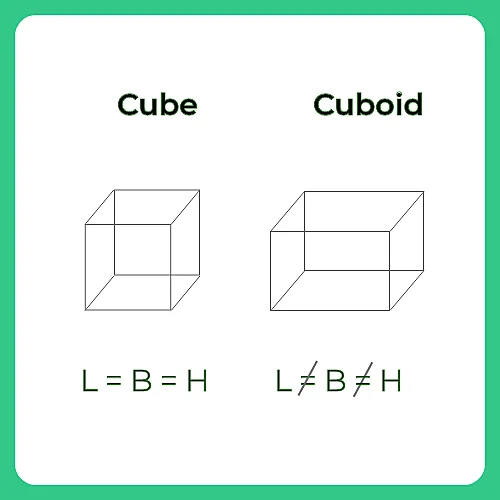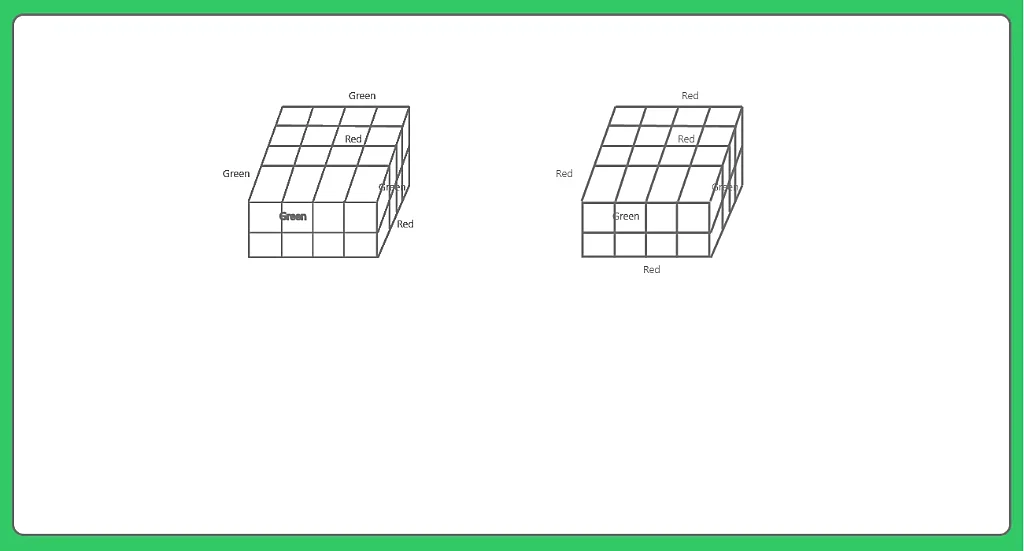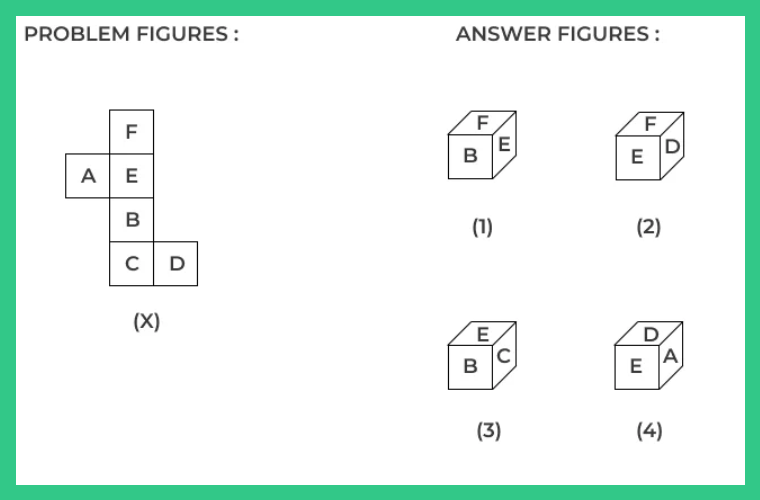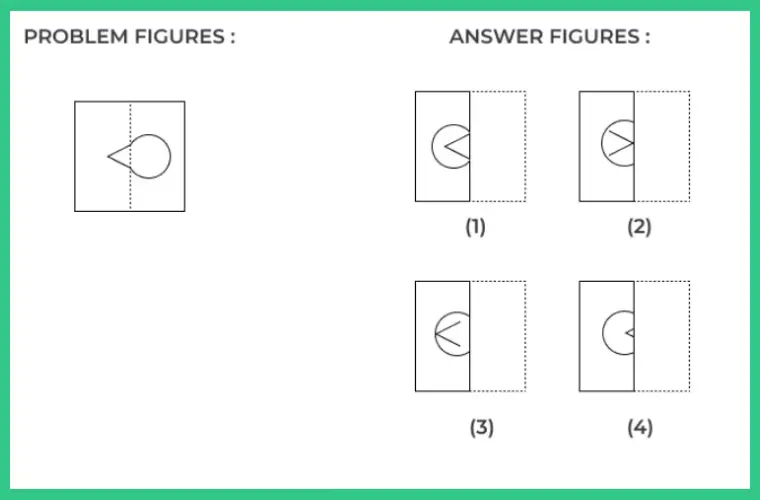# Cube And Cuboid Shapes Formulas

## Cube and Cuboid Formulas :

In this Page Formulas for Cube and Cuboid is given. In cube and Cuboid both Number of Faces , Number of edges and Number of Vertex is same.### Cube:

A cuboid having its length, breadth, height all to be equal in measurement is called as a cube.

A cube is a solid bounded by six square plane regions, where the side of the cube is called edge.

### Formulas for Cube :

• For a cube of side n x n x n painted on all sides which is uniformly cut into smaller cubes of dimension 1 x 1 x 1,
• Number of cubes with 0 side painted= $(n-2) ^{3}$
• Number of cubes with 1 sides painted = $6(n – 2) ^{2}$
• Number of cubes with 2 sides painted = 12(n-2)
• Number of cubes with 3 sides painted= 8(always)

### Cuboid :

All the edges of Cuboid are Not Equal. i.e Measures of Length , breadth and height are not equal.

A Cuboid is made up of six rectangles, each of the rectangle is called the face.

### Formulas for Cuboid :

• For a cuboid of dimension a x b x c painted on all sides which is cut into smaller cubes of dimension 1 x 1 x 1,
• Number of cubes with 0 side painted = (a-2) (b-2) (c-2)
• Number of cubes with 1 sides painted = 2[(a-2) (b-2) + (b-2)(c-2) + (a-2)(c-2) ]
• Number of cubes with 2 sides painted = 4(a+b+c -6)
• Number of cubes with 3 sides painted = 8

### Directions to Solve Cube and Cuboid Formulas

A cube is cut in two equal parts along a plane parallel to one of its faces. One piece is then coloured red on the two larger faces and green on the remaining, while the other is coloured green on two smaller adjacent faces and red on the remaining. Each is then cut into 32 cubes of same size and mixed up.

Question 1. How many cubes have only one coloured face each ?

(A) 32
(B) 8
(C) 16
(D) 0

Explanation:

8 from (I) and 8 from (II)

Therefore 8 from each.Question 2. Choose the box that is similar to the box formed from the given sheet of paper (X).(A) Only 1
(B) Only 2
(C) 1,2,3
(D) 1,3 and 4

Explanation:
When sheet is folded we find A lies Opposite to D , B lies Opposite to F and C lies Opposite to E hence only 2 is correct option.

### Related Banners

Get PrepInsta Prime & get Access to all 200+ courses offered by PrepInsta in One Subscription

### Also Check Out

Question 3.What is the number of cubes with at least one green face each ?

(A) 36
(B) 32
(C) 38
(D) 48

Explanation:

24 from (I) and 14 from (II)Question 4.How many cubes have each one red and another green ?

(A) 0
(B) 8
(C) 16
(D) 24

Explanation:

16 from (I) and 8 from (II)Question 5:The sheet given in the problem figures is a transparent sheet. Choose the correct option which would appear when the transparent sheet is folded at the dotted line.(A) 1
(B) 2
(C) 3
(D) 4